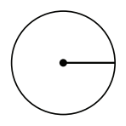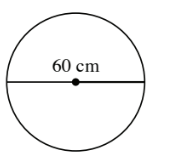### Home > CC2 > Chapter 9 > Lesson 9.2.3 > Problem9-78

9-78.

Find the area of each circle below.

1.radius $=8$ cm

$\text{Area of a circle}=\pi·r^2$

1.$\pi·(30\ \text{cm})^2=900\pi=2827.43\ \text{cm}^2$